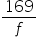Mathematics
Easy

Question

# What is the value of, if f = 13?

## 169    13    -13    -169Hint:

## The correct answer is: 13

### The given expression isThere is one variable and one constant in the above expression. The variables is : ’f’. Value assigned to the variable is are f = 13. We have to substitute this value and find the final value of the expression.There is one term in the above expression. In this term, a constant '169’ is divided by a variable ‘f’We have to first substitute the value and perform the required operations.To substitute the value, we have to replace ‘f' by 13. After substituting the value, we will get the following equation:Therefore, the value of equation is 14So option which is ‘13’ is the right option.

We have to be careful about the operations because of the variables. We have to follow the proper procedure for performing the operations. The variables take different values under different conditions. So we have to be careful while substituting the values. The main part of this type of question is just replacing the variables by their values and performing the operation.

### Related Questions to study#### With Turito Foundation.#### Get an Expert Advice From Turito.